×

Web Demos

Explore the flexibe barcode reading settings to optimize for your specific usage scenario.

WEB TWAIN SDK DEMO

Try the most popular web scanner features: scan images, import local images and PDF files, edit, save to local, upload to database, and etc.

Transform any camera-equipped devices into real-time, browser-based barcode and QR code scanners.

MRZ SCANNER WEB DEMO

Detects the machine-readable zone of a passport, scans the text, and parses into human-readable data.

APP STORE DEMOS

VIEW MORE DEMOS >

# Image Processing 101 Chapter 1.3: Color Space Conversion

May 24, 2019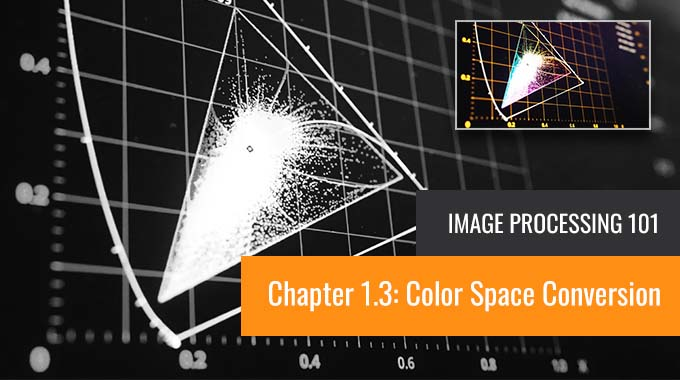In the last post, we discussed a few common color models, specifically RGB, HSV, and YUV. A color model is an abstract mathematical model that describes how colors can be represented as a set of numbers. In this post, we will look at how to convert them to grayscale. Grayscale is a range of gray shades from white to black, as used in a monochrome display or printout. Grayscale images are most commonly used in image processing because smaller data enables developers to do more complex operations in a shorter time.

## 1. Color to Grayscale Conversion

### 1.1 RGB to Grayscale

There are a number of commonly used methods to convert an RGB image to a grayscale image, such as average method and weighted method.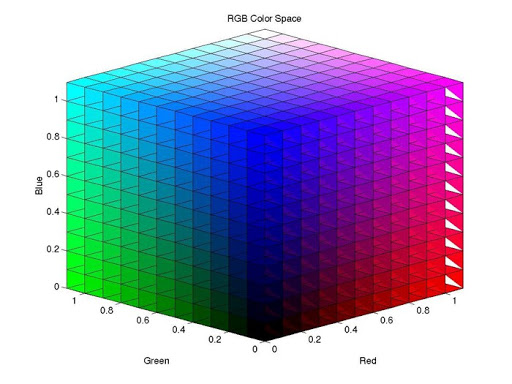#### Average Method

The Average method takes the average value of R, G, and B as the grayscale value.

``````Grayscale = (R + G + B ) / 3
``````

A minor note: Theoretically, the formula is 100% correct. But when writing code, you may encounter uint8 overflow error — the sum of R, G, and B is greater than 255. To avoid the exception, R, G, and B should be calculated, respectively.

``````Grayscale = R / 3 + G / 3 + B / 3
``````

The average method is simple but doesn’t work as well as expected. The reason is that human eyeballs react differently to RGB. Eyes are most sensitive to green light, less sensitive to red light, and the least sensitive to blue light. Therefore, the three colors should have different weights in the distribution. That brings us to the weighted method.

#### The Weighted Method

The weighted method, also called the luminosity method, weighs red, green, and blue according to their wavelengths. The improved formula is as follows:

``````Grayscale = 0.299R + 0.587G + 0.114B
``````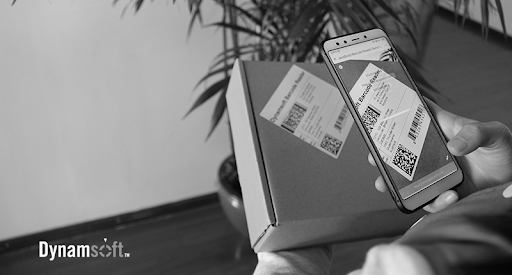### 1.2 YUV to Grayscale

YUV is a color encoding system used for analog television. The YUV color model represents the human perception of color more closely than the standard RGB model used in computer graphics hardware and is more size-efficient.

#### RGB to YUV Conversion

``````Y = 0.299R + 0.587G + 0.114B
U'= (B-Y)*0.565
V'= (R-Y)*0.713
``````

#### The Grayscale Value

The formula for converting RGB to Y is the same as the formula for converting RGB to grayscale. Therefore:

``````Grayscale = Y
``````

### 1.3 HSV to Grayscale

HSV (hue, saturation, value)  and HSL (hue, saturation, lightness or luminance) are transformations of a Cartesian RGB color space.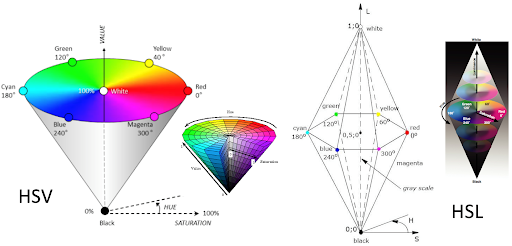To convert HSV to grayscale, we first need to convert HSV to RGB and then convert the RGB triple to a grayscale value.

#### HSV to RGB conversion

``````When 0 ≤ H < 360, 0 ≤ S ≤ 1 and 0 ≤ V ≤ 1:
C = V × S
X = C × (1 - |(H / 60°) mod 2 - 1|)
m = V - C
(R,G,B) = ((R‘+m)×255, (G‘+m)×255, (B‘+m)×255)
``````

#### The Grayscale Value

``````Grayscale = 0.299R + 0.587G + 0.114B
``````

### 1.4 An example of RGB to grayscale using the weighted method

Here is an example of the grayscale conversion method used in a barcode scanner. As we can see in this image, the DataMatrix code on a Thai government lottery has red lines crossed over.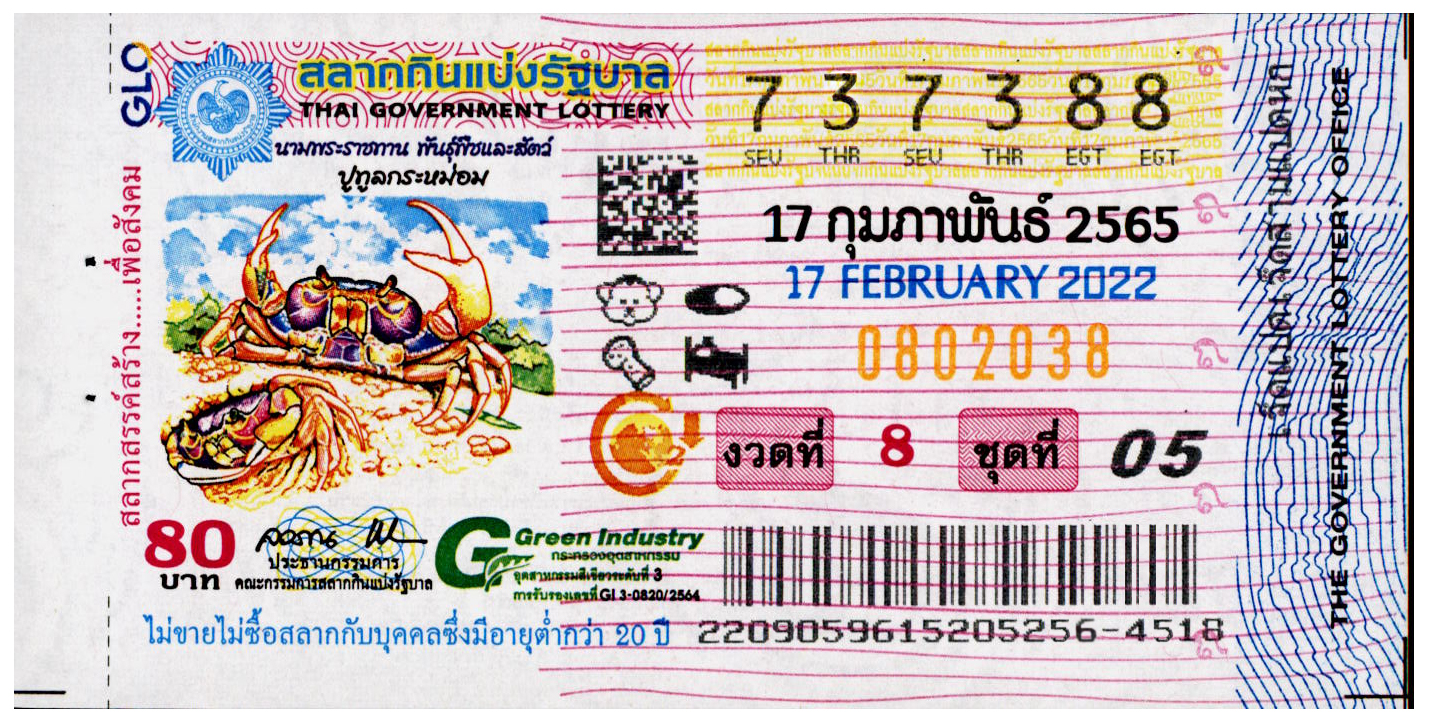The barcode scanner first converts the color image to grayscale and then binary. Using the average method, we get a grayscale image like this one: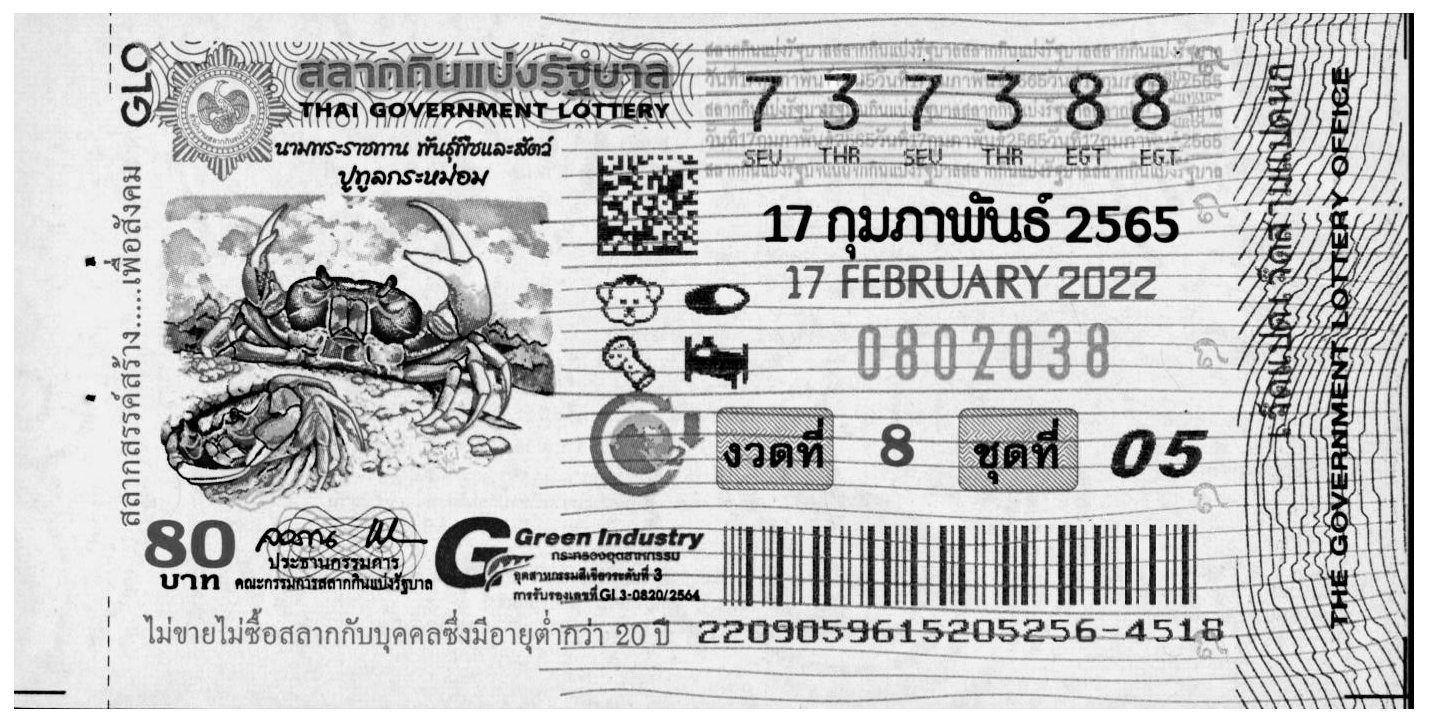To further split the foreground (the DataMatrix code) from the white background with the red lines, let’s give a larger weight to the red channel.

``````Grayscale = 1*Red + 0*Green + 0*Blue
``````

Here is the result. The red lines are lighter compared with the result from the average method, i.e., closer to white.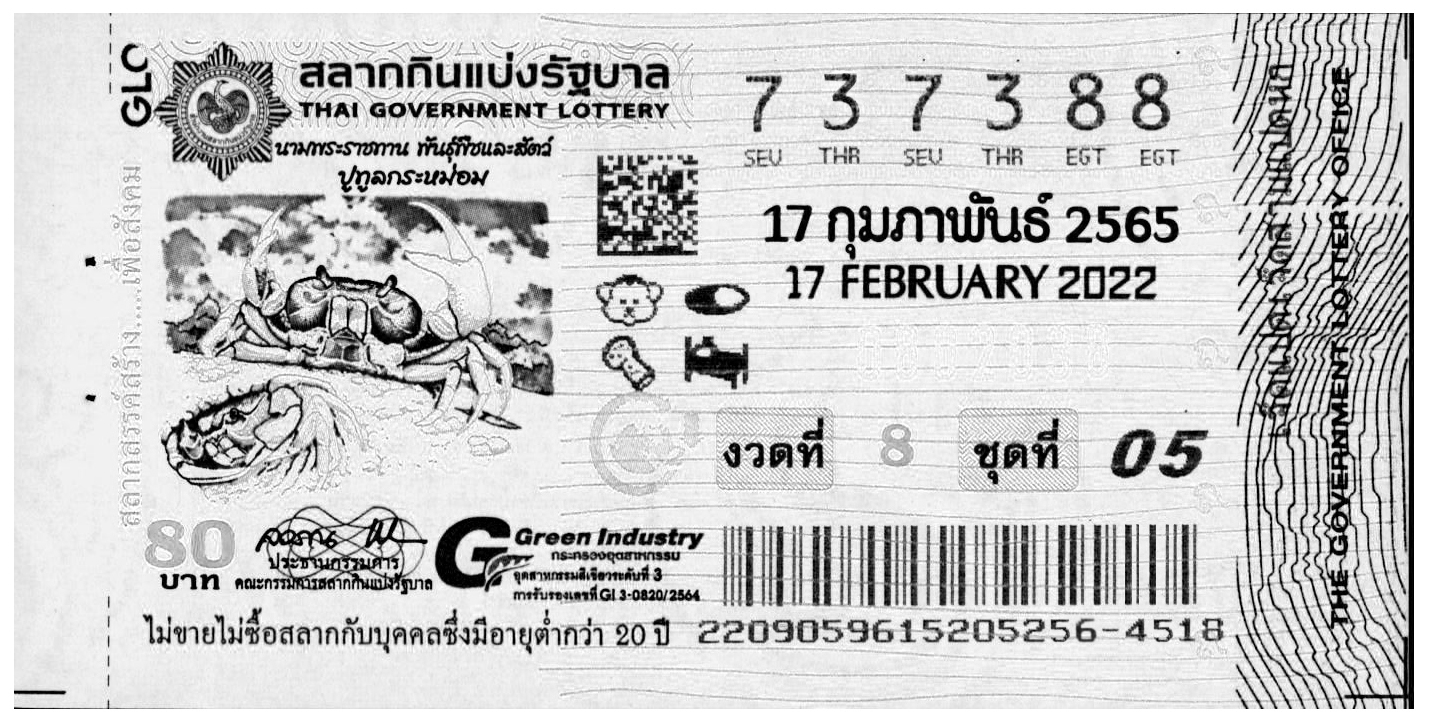Source code used for testing

``````import cv2

blue = RGB_image[:,:,0]
green = RGB_image[:,:,1]
red = RGB_image[:,:,2]
Average_Gray = blue/3+green/3+red/3
Weighted_Gray = (0 * blue) + (0* green) + (1 * red)
``````

## 2. Binarization: Grayscale to black/white Conversion

Binarization converts a grayscale image to a black/white image. This transformation is useful in detecting blobs and further reduces the computational complexity. The critical task is to find a suitable threshold. There are two main methods:

• Local thresholding — calculates the threshold pixel by pixel
• Global thresholding — calculates the threshold once for all pixels

The images below show an example of before and after binarization.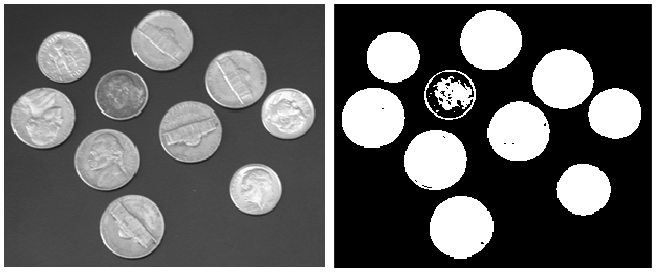### 2.1 Local Thresholding Method

With the local thresholding method, a threshold is calculated at each pixel, which depends on some local statistics such as mean, range, and the variance of the pixel neighborhood. The image is divided into several sub-blocks, and the distribution of gray-value in each block was analyzed.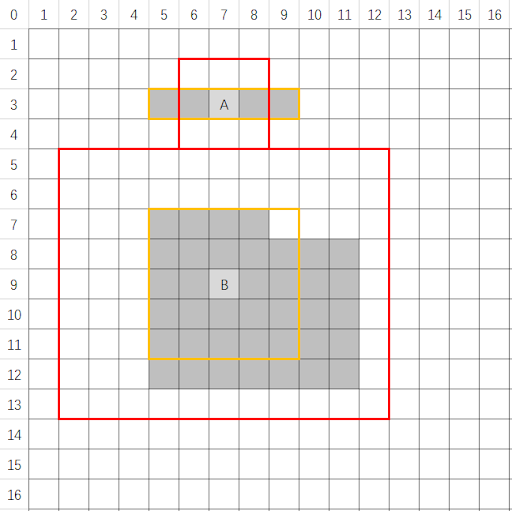### 2.2 Global Thresholding Method

The global thresholding method takes advantage of the image histogram. The image histogram is a statistical graph with grayscale value on the x-axis and the number of pixels for each grayscale on the y-axis.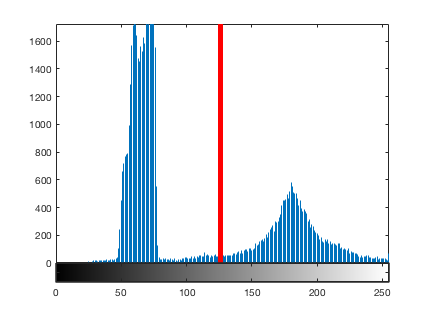Image histogram can be used to automatically determine the value of the threshold to be used for converting a grayscale image to a binary image. The basic idea is to find a point between the peak of the foreground pixel values and the peak of the background pixel values. If the intensity level of a pixel is smaller than the threshold, the pixel is set to black (grayscale = 0). Otherwise, it is set to white (grayscale = 255). The threshold serves as a dividing line.